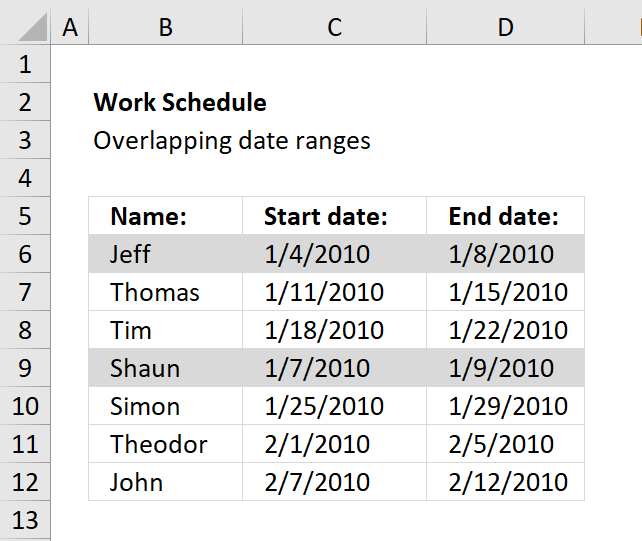Author: Oscar Cronquist Article last updated on November 14, 2018The image above demonstrates a conditional formatting formula that colors a record if there is at least one record that overlaps.

The first and fourth record are highlighted because the date ranges overlap. 1/4/2010 - 1/8/2010 overlaps with 1/7/2010 - 1/9/2010.

Here are the steps needed to create a conditional formatting formula

1. Select cell range B6:D12.
2. Click "Home" tab.
3. Click "Conditional Formatting" button.
4. Click "New Rule..".
5. Click "Use a formula to determine which cells to format".
6. Type =SUMPRODUCT((\$C6<=\$D\$6:\$D\$12)*(\$D6>=\$C\$6:\$C\$12))>1 in "Format values where this formula is TRUE" window.
7. Click "Format.." button.
8. Click "Fill" tab.
9. Pick a color. This color is used to highlight records that overlap.
10. Click "OK".
11. Click "OK".
12. Click "OK".

### Explaining conditional formatting formula

=SUMPRODUCT((\$C6<=\$D\$6:\$D\$12)*(\$D6>=\$C\$6:\$C\$12))>1

Step 1 - Filter records where (\$C6<=\$D\$6:\$D\$12) is TRUE

\$C6<=\$D\$6:\$D\$12 returns an array of TRUE and/or FALSE if date in cell C6 is smaller or equal to each date in \$D\$6:\$D\$12.

All dates in\$D\$6:\$D\$12 are larger than \$C6 so the returning array is (TRUE, TRUE, TRUE,TRUE, TRUE, TRUE,TRUE)

Step 2 - Filter records where (\$D6>=\$C\$6:\$C\$12) is TRUE

(\$D6>=\$C\$6:\$C\$12) returns an array of TRUE and/or FALSE if date in cell D6 is bigger or equal to each date in \$C\$6:\$C\$12.

The returning array is (TRUE, FALSE, FALSE,TRUE, FALSE, FALSE,FALSE)

Step 3 - Putting it all together

(\$C6<=\$D\$6:\$D\$12)* (\$D6>=\$C\$6:\$C\$12)) returns the following array (1,0,0,1,0,0,0).

That means array number one and four is overlapping C6:D6) but in this case we just want to know if any date range is overlapping.

=SUMPRODUCT((\$C6<=\$D\$6:\$D\$12)*(\$D6>=\$C\$6:\$C\$12)) sums the array (1,0,0,1,0,0,0) and returns 2.

If the formula returns a number bigger than one there is at least one overlapping date range.

=SUMPRODUCT((\$C6<=\$D\$6:\$D\$12)*(\$D6>=\$C\$6:\$C\$12))>1 returns TRUE or FALSE.

How to use the SUMPRODUCT function

The SUMPRODUCT function calculates the product of corresponding values and then returns the sum of each multiplication.